### Geometry Fundamentals (2019)

Many geometric techniques and solutions hinge on finding the measures of a shape's angles.

It'll be helpful to think of an angle as the measure of a turning motion or rotation. When we use the unit of degrees to measure angles, one full turn is defined to measure exactly $360$ degrees, which is also written as $360^\circ.$

What is the measure of a half turn?

# Angle Hunt

If you've already taken a geometry class somewhere, you might have been asked to memorize that these little angles created by extending the edges of any polygon will always add up to exactly $360$ degrees.

But, if you think about angles as a "turning" motion, you can really see and understand why that's true.

Using this observation, what is the measure of the the pink-shaded angle above the question mark in the figure?

# Angle Hunt

If this fan of angles adds up to a half turn, what is the value of $x?$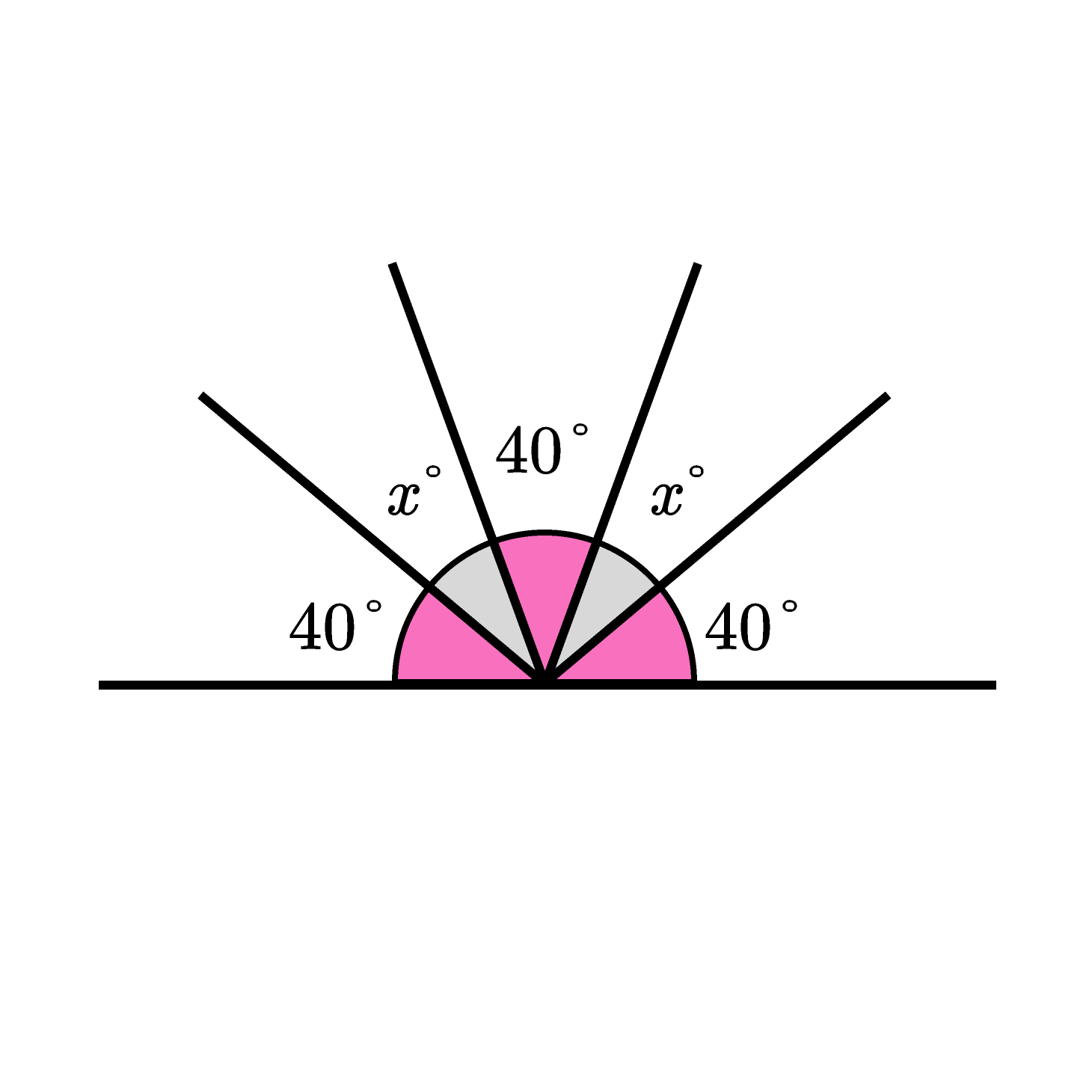# Angle Hunt

Which value is the largest?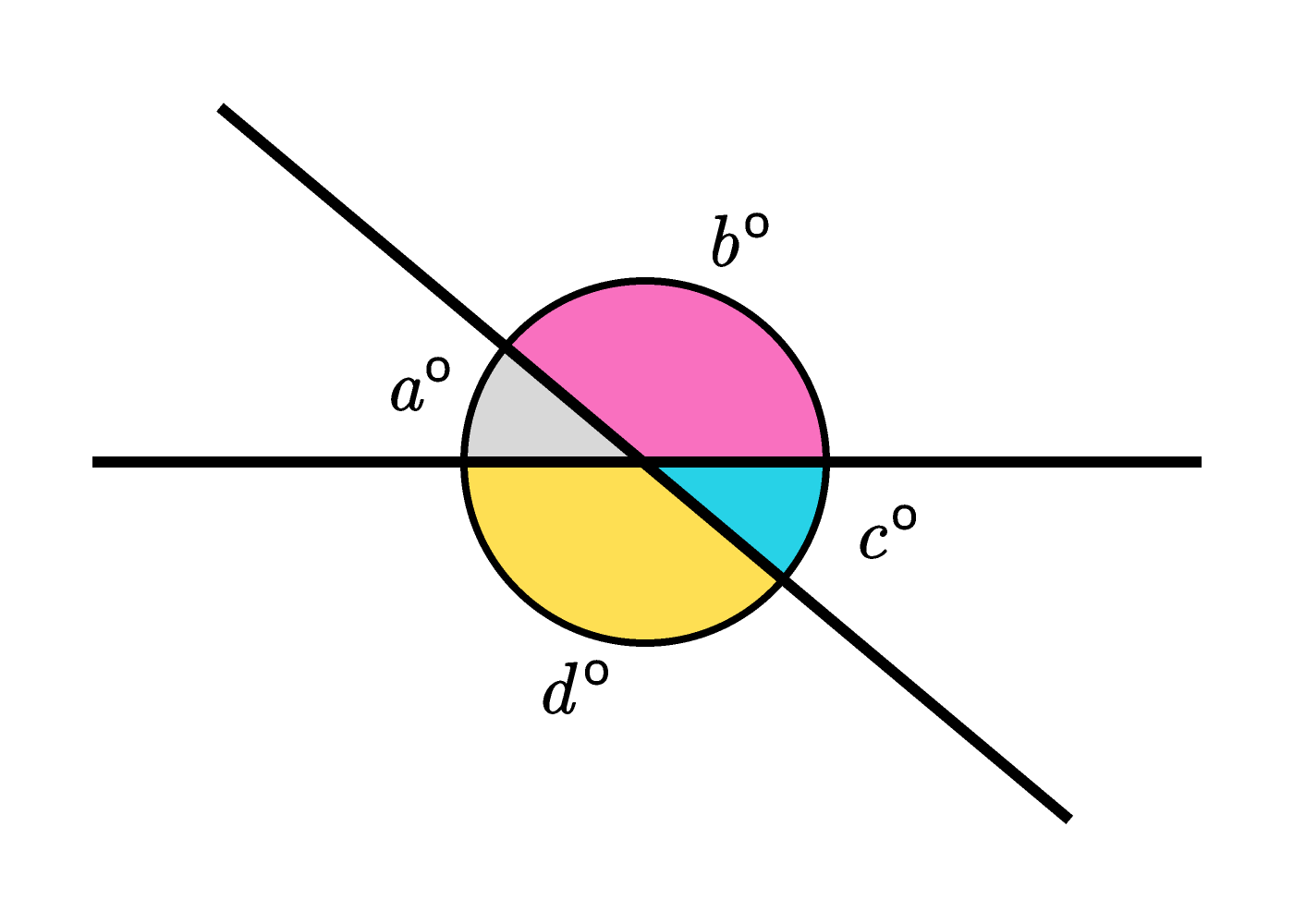# Angle Hunt

The angles $x$ and $y$ are formed by two intersecting lines. What can we say about the measures of this vertical pair of angles?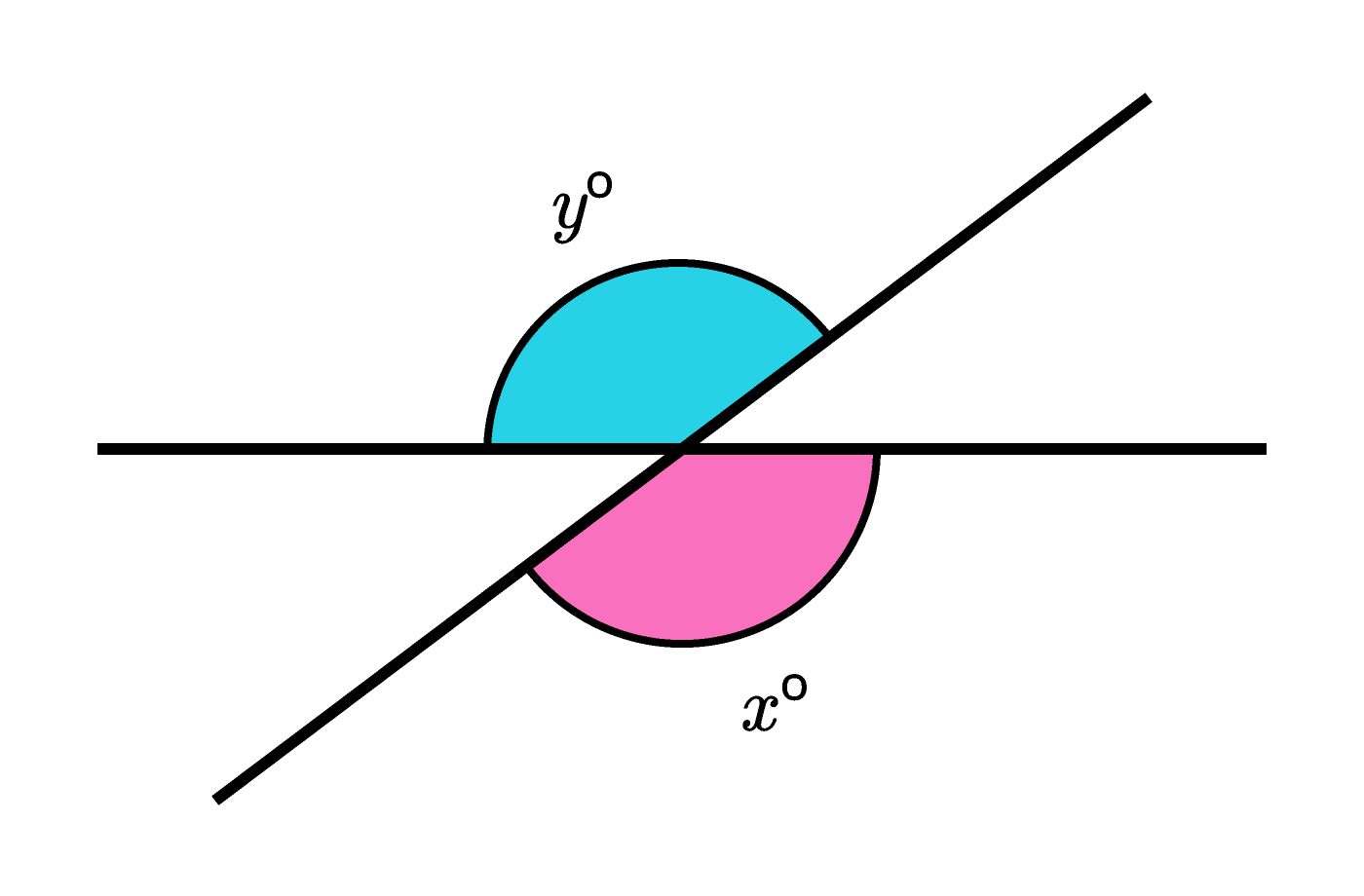# Angle Hunt

A "quarter turn" gets a special name. We call it a right angle, and it has a measure of $90^\circ.$ In diagrams, 90 degree angles are sometimes identified with a small square block instead of a circle-wedge.

# Angle Hunt

What is the value of $x?$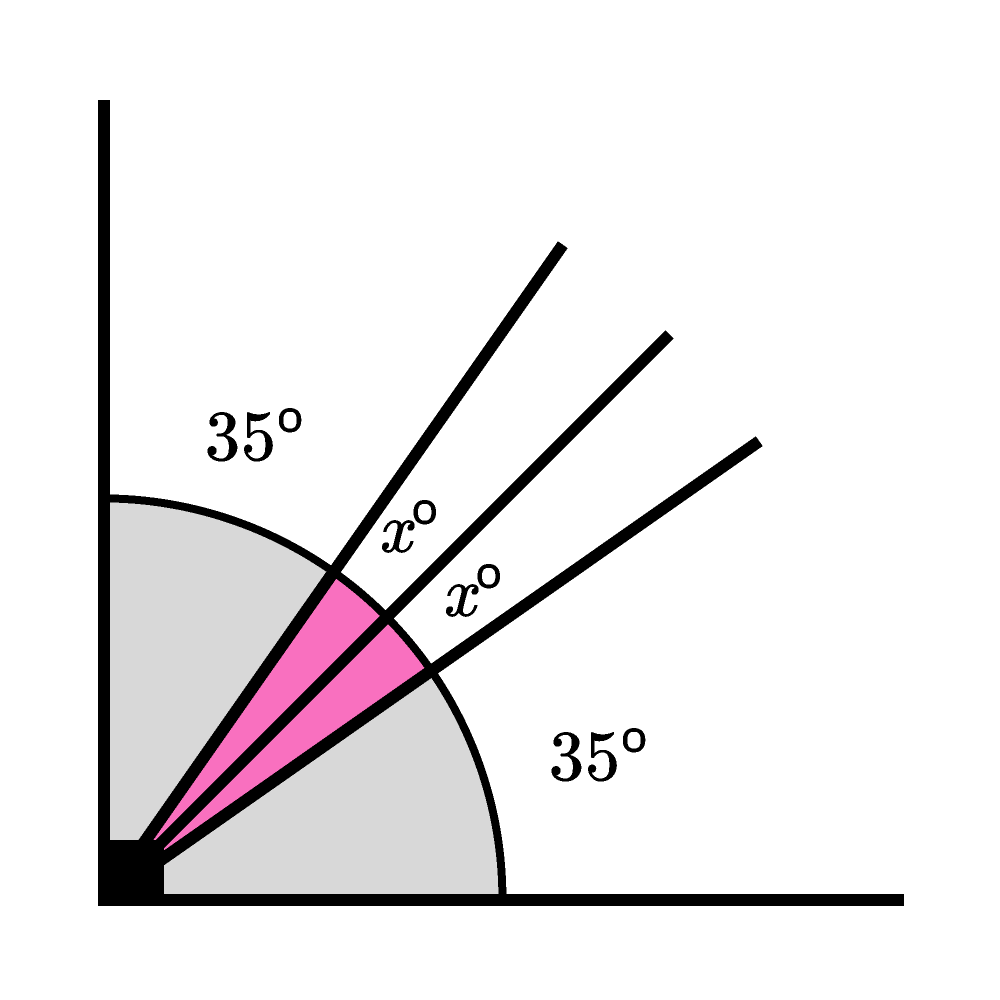# Angle Hunt

Now let's combine using everything that's been introduced so far.

What is the measure of the unknown angle?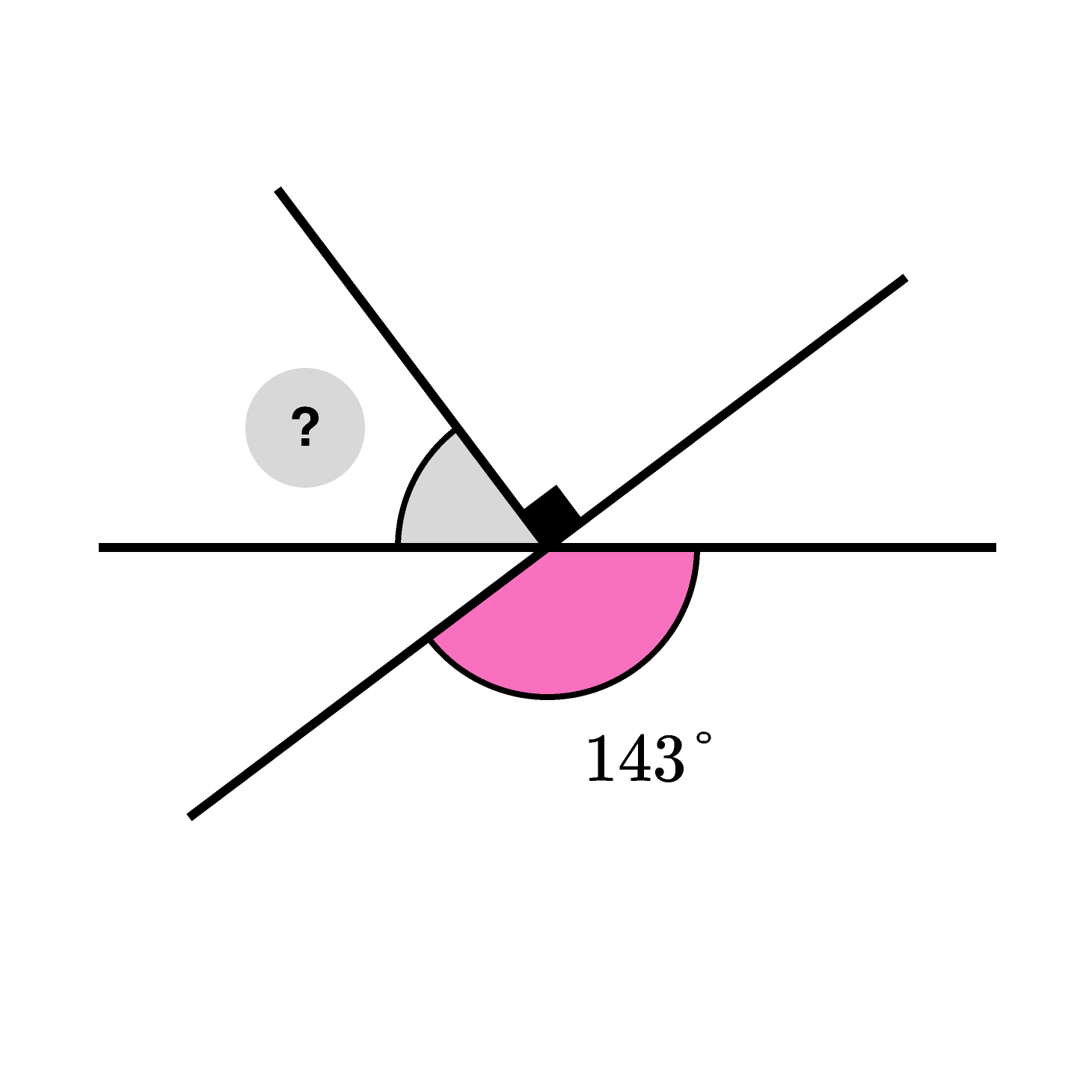# Angle Hunt

×

Problem Loading...

Note Loading...

Set Loading...